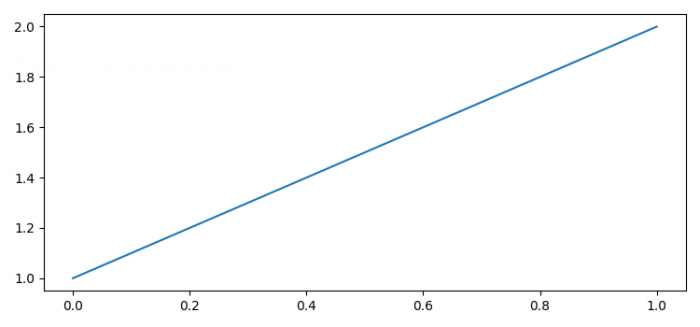# How to convert Matplotlib figure to PIL Image object?

To convert matplotlib figure to PIL image object, we can take the following steps −

• Set the figure size and adjust the padding between and around the subplots.
• Create a new figure or activate an existing figure.
• Plot a list using plot() method.
• Initialize the in-memory buffer.
• Save the buffered image.
• Use PIL image to get the image object.
• Show the current image.
• Close the in-memory I/O buffer.

## Example

import io
from PIL import Image
import matplotlib.pyplot as plt

plt.rcParams["figure.figsize"] = [7.50, 3.50]
plt.rcParams["figure.autolayout"] = True

plt.figure()
plt.plot([1, 2])

img_buf = io.BytesIO()
plt.savefig(img_buf, format='png')

im = Image.open(img_buf)
im.show(title="My Image")

img_buf.close()

## Output